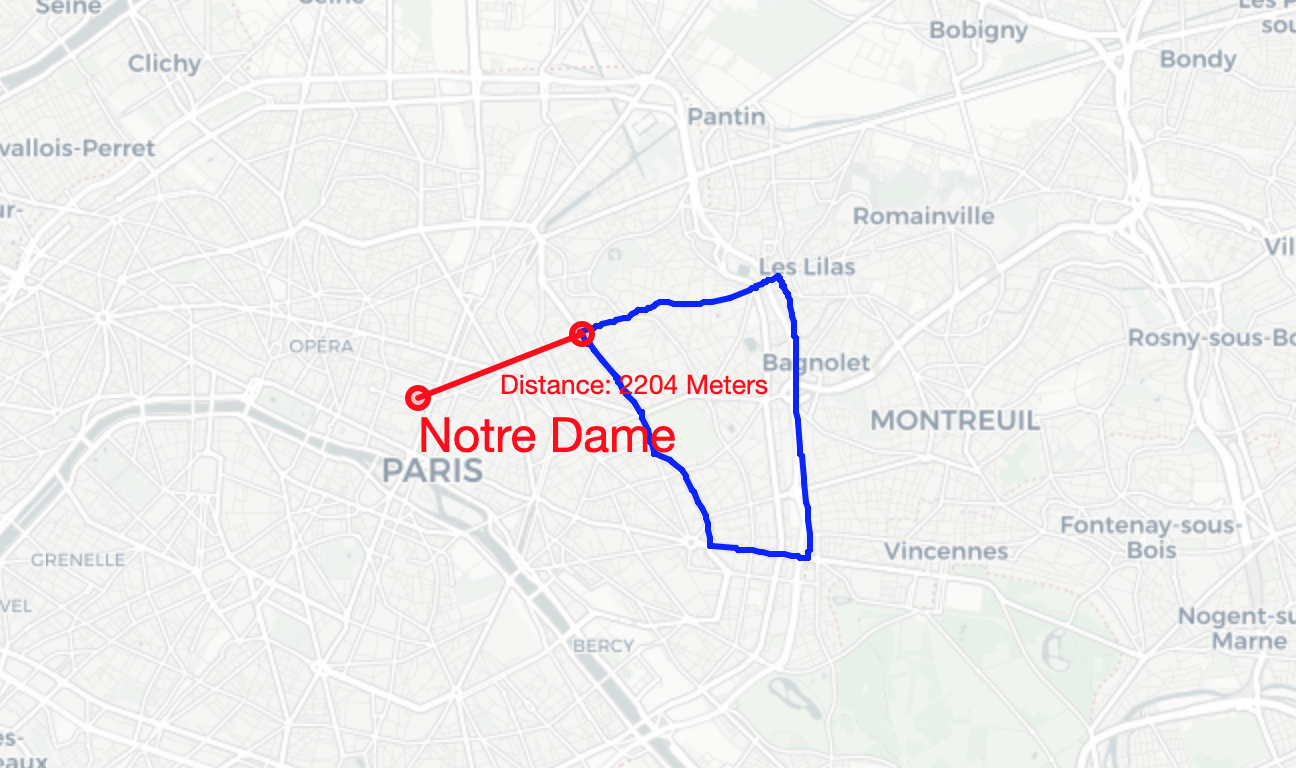# Calculating distances from Points to Polygon Borders in Python — A Paris Example

There are many great packages which help you to work with geospatial data in Python. However, if you need to customize, it can get tricky.

I want to show how to calculate distances in meters between latitude, longitude pairs and polygons. Consider that I don’t want to calculate the distance to the midpoint of the polygon, but to the closest border.

I will show this using the following example: how far is Notre Dame away from every Arrondissement?

All code is available in my Git. I will include links to relevant functions instead of writing out the syntax in this post.

# Setting up your Environment and getting the Data

For visualisation we will use the `folium` library in python. For geometries we will use `geopy`. Install the packages with:

`pip install folium geopy`

I want to show the methodology using arrondissements data from Paris. Please download the GeoJSON file from https://www.data.gouv.fr/en/datasets/arrondissements-1/#_ and save the file in your project root directory.

Let’s now have a look at the arrondissement as well as the location of Notre Dame in Paris.

That’s the code to generate this map.

`import foliumfrom folium.features import DivIconimport geojsonwith open('arrondissements.geojson') as f:    data = geojson.loads(f.read())# Set your point of interest. In our case that is Notre Dame.poi_name = 'Notre Dam'poi = [48.864716, 2.349014]# Map it!m = folium.Map(location=poi, zoom_start=12, tiles='cartodbpositron')# Display Arrondissementsfolium.GeoJson(data).add_to(m)# Display Arrondissements Numbersfor arrond in data['features']:    loc = arrond['properties']['geom_x_y']    nr = arrond['properties']['c_ar']    folium.map.Marker(location=loc, icon=DivIcon(        icon_size=(150, 36),        icon_anchor=(0, 0),        html='<div style="font-size: 15pt; color:rgb(41,111,255)">{}</div>'.format(str(nr))    )).add_to(m)# Display POIfolium.CircleMarker(location=poi, color='red', radius=5, fill='red').add_to(m)# Display POI Namefolium.map.Marker(location=poi, icon=DivIcon(    icon_size=(150, 36),    icon_anchor=(0, 0),    html='<div style="font-size: 18pt; color: red">{}</div>'.format(poi_name))).add_to(m)m.save('map_1.html')`

# Calculating the Distance to one Arrondissement

Whereas Python is great with calculating distances in Cartesian Coordinate Systems, some workarounds are required if you are working with geospatial data.

1. Outputs of libraries need to be converted in usable metrics. See @jbndir reply here.
2. Distance calculations need to take into account the spherical form of the earth. We can use the Haversine formula to calculate great circle distance as outlined here. (Note that this becomes increasingly important for big distances)

## Another Challenge — Measuring the distance to what?

We want to measure the distance of a point to a polygon. But a polygon can be separated in various line segments and points. Should we measure the distance to lines, points or both?

Let’s look at a simple example:

We have a line between A and B and want to calculate the minimum distance to C. As we all know, the shortest distance is the orthogonal projection onto the line AB and the closest point on the line is X.

However in the following simple example, we are not able to project an orthogonal line, which is why the minimum distance is to the closest point.

## Middle Ground — Measure the distance to the Midpoint!

In order to keep our algorithm lean, let’s not account for these specific cases and always calculate the distance to the middle point of our lines. Especially in accurately defined polygons (on a small space), the differences are negligible.

Let’s follow the midpoint calculation outlined here and the implementation in Python here (thanks, @nelfin).

`def midpoint(a, b):... Please find the function here.`

Having the midpoint, we can now calculate the distance to a point:

`def calculate_dist_to_line(line_a_lat, line_a_lng, line_b_lat, line_b_lng, point_object):...Please find the function here.`

Now, a polygon consists of many different lines, which is why we need to loop through all lines and find the one with the lowest distance:

`def get_min_distance_to_arr(arr_coords, point_object, unit='m'):...Please find the function here.`

## Nice! Let’s look at one Arrondissement now

Using our functions, we can easily get the minimum distance and the PolyLine for any Arrondissement:

`# Example with Arrondissement 20coords = [x['geometry']['coordinates'] for x in data['features'] if x['properties']['c_ar'] == 20]p = Point(latitude=poi,longitude=poi)distance, line = fu.get_min_distance_to_arr(arr_coords=coords,point_object=p)line_midpoint = fu.get_line_midpoint(line)`

The plot also looks good — and accurate!The shortest distance from Notre Dame to the border of Arrondissement 20.

The code:

`# Map it!m = folium.Map(location=poi, zoom_start=12, tiles='cartodbpositron')# Display POIfolium.CircleMarker(location=poi, color='red', radius=5, fill='red').add_to(m)# Display POI Namefolium.map.Marker(location=poi, icon=DivIcon(    icon_size=(150, 36),    icon_anchor=(0, 0),    html='<div style="font-size: 18pt; color: red">{}</div>'.format(poi_name))).add_to(m)for i, _ in enumerate(coords):    if i+1 < len(coords):        folium.PolyLine(locations=[(coords[i],coords[i]),(coords[i+1],coords[i+1])], color='blue').add_to(m)    else:        folium.PolyLine(locations=[(coords[i], coords[i]), (coords, coords)],                        color='blue').add_to(m)folium.PolyLine(locations=line, color='red').add_to(m)folium.PolyLine(locations=[poi,[line_midpoint.latitude,line_midpoint.longitude]], color='red').add_to(m)folium.CircleMarker(location=[line_midpoint.latitude,line_midpoint.longitude], color='red', radius=5, fill='red').add_to(m)new_line_mp = fu.get_line_midpoint([poi,[line_midpoint.latitude,line_midpoint.longitude]])folium.map.Marker(location=[new_line_mp.latitude,new_line_mp.longitude], icon=DivIcon(    icon_size=(150, 36),    icon_anchor=(0, 0),    html='<div style="font-size: 10pt; color: red">Distance: {} Meters</div>'.format(round(dist)))).add_to(m)m.save('map_2.html')`

## Let’s do this with all other Arrondissements!

Since we have the basic logic ready, it’s easy to loop through it and plot all data points together.

If you’re interested in finding out which arrondissement is closest, use the output of the function get_min_distance_to_arr!

The map doesn’t look pretty, but is correct :)

That’s the code:

`# Map all!m = folium.Map(location=poi, zoom_start=12, tiles='cartodbpositron')# Display POIfolium.CircleMarker(location=poi, color='red', radius=5, fill='red').add_to(m)# Display POI Namefolium.map.Marker(location=poi, icon=DivIcon(    icon_size=(150, 36),    icon_anchor=(0, 0),    html='<div style="font-size: 18pt; color: red">{}</div>'.format(poi_name))).add_to(m)# Display Arrondissementsfolium.GeoJson(data).add_to(m)# Display Arrondissements Numbersfor arrond in data['features']:    loc = arrond['properties']['geom_x_y']    nr = arrond['properties']['c_ar']    folium.map.Marker(location=loc, icon=DivIcon(        icon_size=(150, 36),        icon_anchor=(0, 0),        html='<div style="font-size: 15pt; color:rgb(41,111,255)">{}</div>'.format(str(nr))    )).add_to(m)for arrond in data['features']:    coords = arrond['geometry']['coordinates']    p = Point(latitude=poi,longitude=poi)    dist, line = fu.get_min_distance_to_arr(arr_coords=coords,point_object=p)    line_midpoint = fu.get_line_midpoint(line)    # for i, _ in enumerate(coords):    #     if i+1 < len(coords):    #         folium.PolyLine(locations=[(coords[i],coords[i]),(coords[i+1],coords[i+1])], color='blue').add_to(m)    #     else:    #         folium.PolyLine(locations=[(coords[i], coords[i]), (coords, coords)],    #                         color='blue').add_to(m)    folium.PolyLine(locations=line, color='red').add_to(m)    folium.PolyLine(locations=[poi,[line_midpoint.latitude,line_midpoint.longitude]], color='red').add_to(m)    folium.CircleMarker(location=[line_midpoint.latitude,line_midpoint.longitude], color='red', radius=5, fill='red').add_to(m)    new_line_mp = fu.get_line_midpoint([poi,[line_midpoint.latitude,line_midpoint.longitude]])    folium.map.Marker(location=[line_midpoint.latitude,line_midpoint.longitude], icon=DivIcon(        icon_size=(150, 36),        icon_anchor=(0, 0),        html='<div style="font-size: 10pt; color: red">Distance: {} Meters</div>'.format(round(dist))    )).add_to(m)m.save('map_3.html')`

Reach out with any questions you might have!

## Analytics Vidhya

Analytics Vidhya is a community of Analytics and Data…## Analytics Vidhya

Analytics Vidhya is a community of Analytics and Data Science professionals. We are building the next-gen data science ecosystem https://www.analyticsvidhya.com